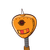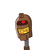# The sum of two integers is -56. If one of them is 35, find the other

The sum of two integers is -56. If one of them is 35,
find the other

### 2 thoughts on “The sum of two integers is -56. If one of them is 35,<br /> find the other”

1.-91

Step-by-step explanation:

Let x be the unknown integer.

we have,

35+x = -56

x = -56-35

= -91

Hence -91 is the other integer

2.